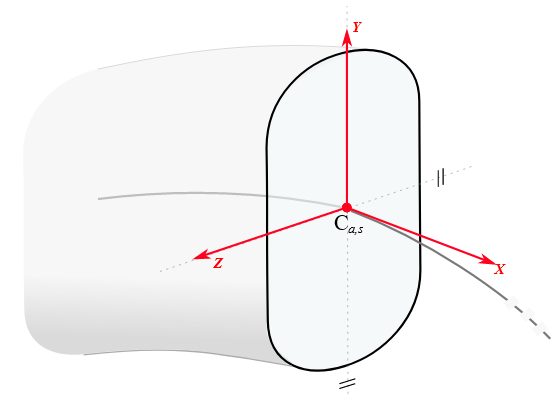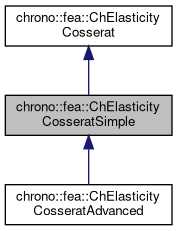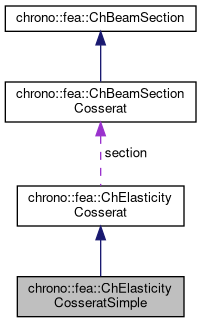chrono::fea::ChElasticityCosseratSimple Class Reference

## Description

Simple linear elasticity model for a Cosserat beam, using basic material properties (zz and yy moments of inertia, area, Young modulus, etc.).

Uniform stiffness properties E,G are hence assumed through the section. The classical Timoshenko beam theory is encompassed in this model, that can be interpreted as a 3D extension of the Timoshenko beam theory. This can be shared between multiple beams.#include <ChBeamSectionCosserat.h>

Inheritance diagram for chrono::fea::ChElasticityCosseratSimple:[legend]
Collaboration diagram for chrono::fea::ChElasticityCosseratSimple:[legend]

## Public Member Functions

ChElasticityCosseratSimple (const double mIyy, const double mIzz, const double mJ, const double mG, const double mE, const double mA, const double mKs_y, const double mKs_z)

void SetArea (double ma)
Set the A area of the beam.

double GetArea () const

void SetIyy (double ma)
Set the Iyy second moment of area of the beam (for bending about y in xz plane), defined as $$I_y = \int_\Omega z^2 dA$$. More...

double GetIyy () const

void SetIzz (double ma)
Set the Izz second moment of area of the beam (for bending about z in xy plane). More...

double GetIzz () const

void SetJ (double ma)
Set the J torsion constant of the beam (for torsion about x axis)

double GetJ () const

void SetKsy (double ma)
Set the Timoshenko shear coefficient Ks for y shear, usually about 0.8, (for elements that use this, ex. More...

double GetKsy () const

void SetKsz (double ma)
Set the Timoshenko shear coefficient Ks for z shear, usually about 0.8, (for elements that use this, ex. More...

double GetKsz () const

virtual void SetAsRectangularSection (double width_y, double width_z)
Shortcut: set Area, Ixx, Iyy, Ksy, Ksz and J torsion constant at once, given the y and z widths of the beam assumed with rectangular shape. More...

virtual void SetAsCircularSection (double diameter)
Shortcut: set Area, Ixx, Iyy, Ksy, Ksz and J torsion constant at once, given the diameter of the beam assumed with circular shape. More...

void SetYoungModulus (double mE)
Set E, the Young elastic modulus (N/m^2)

double GetYoungModulus () const

void SetGshearModulus (double mG)
Set G, the shear modulus.

double GetGshearModulus () const

void SetGwithPoissonRatio (double mpoisson)
Set G, the shear modulus, given current E and the specified Poisson ratio.

virtual void ComputeStress (ChVector<> &stress_n, ChVector<> &stress_m, const ChVector<> &strain_e, const ChVector<> &strain_k) override
Compute the generalized cut force and cut torque. More...

virtual void ComputeStiffnessMatrix (ChMatrixNM< double, 6, 6 > &K, const ChVector<> &strain_e, const ChVector<> &strain_k) override
Compute the 6x6 tangent material stiffness matrix [Km] = dσ/dε. More...

## Public Attributes

double Iyy

double Izz

double J

double G

double E

double A

double Ks_y

double Ks_zPublic Attributes inherited from chrono::fea::ChElasticityCosserat
ChBeamSectionCosseratsection

## ◆ ChElasticityCosseratSimple()

 chrono::fea::ChElasticityCosseratSimple::ChElasticityCosseratSimple ( const double mIyy, const double mIzz, const double mJ, const double mG, const double mE, const double mA, const double mKs_y, const double mKs_z )
inline
Parameters
 mIyy Iyy second moment of area of the beam $$I_y = \int_\Omega z^2 dA$$ mIzz Izz second moment of area of the beam $$I_z = \int_\Omega y^2 dA$$ mJ torsion constant (torsion rigidity will be G*J, torsional stiffness = G*J*length) mG G shear modulus mE E young modulus mA A area mKs_y Timoshenko shear coefficient Ks for y shear mKs_z Timoshenko shear coefficient Ks for z shear

## ◆ ComputeStiffnessMatrix()

 void chrono::fea::ChElasticityCosseratSimple::ComputeStiffnessMatrix ( ChMatrixNM< double, 6, 6 > & K, const ChVector<> & strain_e, const ChVector<> & strain_k )
overridevirtual

Compute the 6x6 tangent material stiffness matrix [Km] = dσ/dε.

Parameters
 K 6x6 stiffness matrix strain_e local strain (deformation part): x= elongation, y and z are shear strain_k local strain (curvature part), x= torsion, y and z are line curvatures

Reimplemented from chrono::fea::ChElasticityCosserat.

## ◆ ComputeStress()

 void chrono::fea::ChElasticityCosseratSimple::ComputeStress ( ChVector<> & stress_n, ChVector<> & stress_m, const ChVector<> & strain_e, const ChVector<> & strain_k )
overridevirtual

Compute the generalized cut force and cut torque.

Parameters
 stress_n local stress (generalized force), x component = traction along beam stress_m local stress (generalized torque), x component = torsion torque along beam strain_e local strain (deformation part): x= elongation, y and z are shear strain_k local strain (curvature part), x= torsion, y and z are line curvatures

Implements chrono::fea::ChElasticityCosserat.

## ◆ SetAsCircularSection()

 void chrono::fea::ChElasticityCosseratSimple::SetAsCircularSection ( double diameter )
virtual

Shortcut: set Area, Ixx, Iyy, Ksy, Ksz and J torsion constant at once, given the diameter of the beam assumed with circular shape.

You must set E and G anyway.

## ◆ SetAsRectangularSection()

 void chrono::fea::ChElasticityCosseratSimple::SetAsRectangularSection ( double width_y, double width_z )
virtual

Shortcut: set Area, Ixx, Iyy, Ksy, Ksz and J torsion constant at once, given the y and z widths of the beam assumed with rectangular shape.

You must set E and G anyway.

## ◆ SetIyy()

 void chrono::fea::ChElasticityCosseratSimple::SetIyy ( double ma )
inline

Set the Iyy second moment of area of the beam (for bending about y in xz plane), defined as $$I_y = \int_\Omega z^2 dA$$.

Note: some textbook calls this Iyy as Iy Ex SI units: [m^4]

## ◆ SetIzz()

 void chrono::fea::ChElasticityCosseratSimple::SetIzz ( double ma )
inline

Set the Izz second moment of area of the beam (for bending about z in xy plane).

defined as $$I_z = \int_\Omega y^2 dA$$. Note: some textbook calls this Izz as Iz Ex SI units: [m^4]

## ◆ SetKsy()

 void chrono::fea::ChElasticityCosseratSimple::SetKsy ( double ma )
inline

Set the Timoshenko shear coefficient Ks for y shear, usually about 0.8, (for elements that use this, ex.

the Timoshenko beams, or Reddy's beams)

## ◆ SetKsz()

 void chrono::fea::ChElasticityCosseratSimple::SetKsz ( double ma )
inline

Set the Timoshenko shear coefficient Ks for z shear, usually about 0.8, (for elements that use this, ex.

the Timoshenko beams, or Reddy's beams)

The documentation for this class was generated from the following files:
• /builds/uwsbel/chrono/src/chrono/fea/ChBeamSectionCosserat.h
• /builds/uwsbel/chrono/src/chrono/fea/ChBeamSectionCosserat.cpp